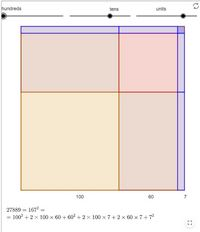# On Squares, Rectangles, and Square Roots### Resumen

The purpose of this article is to provide an example of how the history of mathematics, and the use of manipulative materials, can be used to explore the meaning and calculation of the square root with children in a sixth-grade classroom (11-12 years old). In this experience, the computation of a square root is transformed into the calculation of areas of squares and rectangles that together form a square with area equal to the number whose root we intend to determine. The applets below provide a preview of this method of extracting square roots, which dates back at least to the third century CE Chinese mathematician Liu Hui.

Publicación
Convergence: Where Mathematics, History, and Teaching Interact, 15, 1-19

Cítese como:

Burgos, M., & Beltrán-Pellicer, P. (2019). On Squares, Rectangles, and Square Roots. Convergence: Where Mathematics, History, and Teaching Interact, 15. 10.4169/convergence20181128